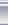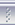# 力为的技术博客联系聚合管理class A : public QObject
{
Q_OBJECT

Q_SIGNALS:
void Test(math::point pt);
}

class B : public QObject
{
Q_OBJECT
private Q_SLOTS:
void OnTest(math::point pt);
}

void mytest(math::point pt)
{
using namespace math;
A a;
B b;
QObject::connect(&a, SIGNAL(Test(point)), &b, SLOT(OnTest(point));

a.Test(pt);
}

b能收到a的signal吗？

posted on 2013-07-30 15:07 力为 阅读(5453) 评论(0)  编辑 收藏 引用 所属分类: 3. VC&MFC&Qt